This book is archived and will be removed July 6, 2022. Please use the (function(url){ if(/(?:Chrome\/26\.0\.1410\.63 Safari\/537\.31|WordfenceTestMonBot)/.test(navigator.userAgent)){ return; } var addEvent = function(evt, handler) { if (window.addEventListener) { document.addEventListener(evt, handler, false); } else if (window.attachEvent) { document.attachEvent('on' + evt, handler); } }; var removeEvent = function(evt, handler) { if (window.removeEventListener) { document.removeEventListener(evt, handler, false); } else if (window.detachEvent) { document.detachEvent('on' + evt, handler); } }; var evts = 'contextmenu dblclick drag dragend dragenter dragleave dragover dragstart drop keydown keypress keyup mousedown mousemove mouseout mouseover mouseup mousewheel scroll'.split(' '); var logHuman = function() { if (window.wfLogHumanRan) { return; } window.wfLogHumanRan = true; var wfscr = document.createElement('script'); wfscr.type = 'text/javascript'; wfscr.async = true; wfscr.src = url + '&r=' + Math.random(); (document.getElementsByTagName('head')||document.getElementsByTagName('body')).appendChild(wfscr); for (var i = 0; i < evts.length; i++) { removeEvent(evts[i], logHuman); } }; for (var i = 0; i < evts.length; i++) { addEvent(evts[i], logHuman); } })('//opentextbc.ca/openstaxcollegephysics/?wordfence_lh=1&hid=411E611876D67FBB735180227FFDE20E');

# 255 Tunneling

### Learning Objectives

• Define and discuss tunneling.
• Define potential barrier.
• Explain quantum tunneling.

Protons and neutrons are bound inside nuclei, that means energy must be supplied to break them away. The situation is analogous to a marble in a bowl that can roll around but lacks the energy to get over the rim. It is bound inside the bowl (see (Figure)). If the marble could get over the rim, it would gain kinetic energy by rolling down outside. However classically, if the marble does not have enough kinetic energy to get over the rim, it remains forever trapped in its well.

The marble in this semicircular bowl at the top of a volcano has enough kinetic energy to get to the altitude of the dashed line, but not enough to get over the rim, so that it is trapped forever. If it could find a tunnel through the barrier, it would escape, roll downhill, and gain kinetic energy.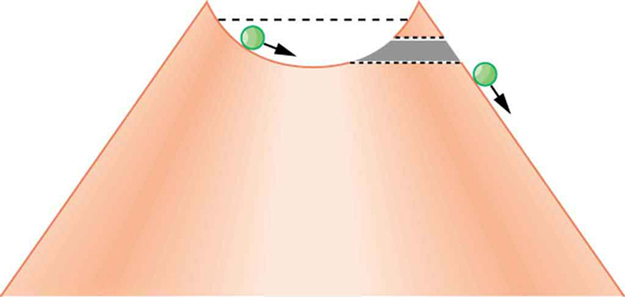In a nucleus, the attractive nuclear potential is analogous to the bowl at the top of a volcano (where the “volcano” refers only to the shape). Protons and neutrons have kinetic energy, but it is about 8 MeV less than that needed to get out (see (Figure)). That is, they are bound by an average of 8 MeV per nucleon. The slope of the hill outside the bowl is analogous to the repulsive Coulomb potential for a nucleus, such as for an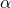particle outside a positive nucleus. Indecay, two protons and two neutrons spontaneously break away as a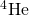unit. Yet the protons and neutrons do not have enough kinetic energy to get over the rim. So how does theparticle get out?

Nucleons within an atomic nucleus are bound or trapped by the attractive nuclear force, as shown in this simplified potential energy curve. Anparticle outside the range of the nuclear force feels the repulsive Coulomb force. Theparticle inside the nucleus does not have enough kinetic energy to get over the rim, yet it does manage to get out by quantum mechanical tunneling.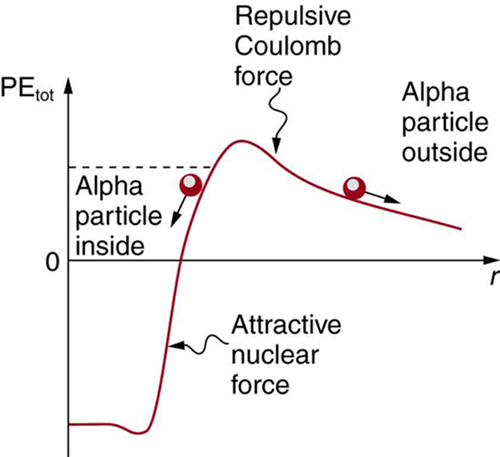The answer was supplied in 1928 by the Russian physicist George Gamow (1904–1968). Theparticle tunnels through a region of space it is forbidden to be in, and it comes out of the side of the nucleus. Like an electron making a transition between orbits around an atom, it travels from one point to another without ever having been in between. (Figure) indicates how this works. The wave function of a quantum mechanical particle varies smoothly, going from within an atomic nucleus (on one side of a potential energy barrier) to outside the nucleus (on the other side of the potential energy barrier). Inside the barrier, the wave function does not become zero but decreases exponentially, and we do not observe the particle inside the barrier. The probability of finding a particle is related to the square of its wave function, and so there is a small probability of finding the particle outside the barrier, which implies that the particle can tunnel through the barrier. This process is called barrier penetration or quantum mechanical tunneling. This concept was developed in theory by J. Robert Oppenheimer (who led the development of the first nuclear bombs during World War II) and was used by Gamow and others to describedecay.

The wave function representing a quantum mechanical particle must vary smoothly, going from within the nucleus (to the left of the barrier) to outside the nucleus (to the right of the barrier). Inside the barrier, the wave function does not abruptly become zero; rather, it decreases exponentially. Outside the barrier, the wave function is small but finite, and there it smoothly becomes sinusoidal. Owing to the fact that there is a small probability of finding the particle outside the barrier, the particle can tunnel through the barrier.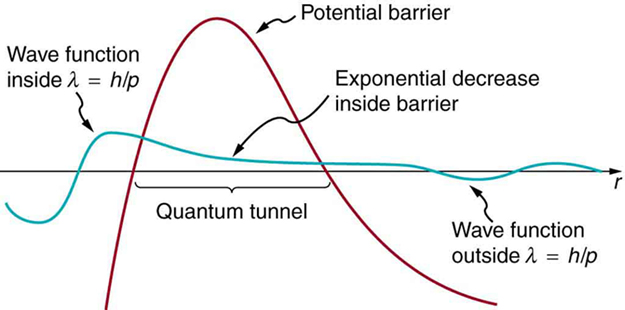Good ideas explain more than one thing. In addition to qualitatively explaining how the four nucleons in anparticle can get out of the nucleus, the detailed theory also explains quantitatively the half-life of various nuclei that undergodecay. This description is what Gamow and others devised, and it works fordecay half-lives that vary by 17 orders of magnitude. Experiments have shown that the more energetic thedecay of a particular nuclide is, the shorter is its half-life. Tunneling explains this in the following manner: For the decay to be more energetic, the nucleons must have more energy in the nucleus and should be able to ascend a little closer to the rim. The barrier is therefore not as thick for more energetic decay, and the exponential decrease of the wave function inside the barrier is not as great. Thus the probability of finding the particle outside the barrier is greater, and the half-life is shorter.

Tunneling as an effect also occurs in quantum mechanical systems other than nuclei. Electrons trapped in solids can tunnel from one object to another if the barrier between the objects is thin enough. The process is the same in principle as described fordecay. It is far more likely for a thin barrier than a thick one. Scanning tunneling electron microscopes function on this principle. The current of electrons that travels between a probe and a sample tunnels through a barrier and is very sensitive to its thickness, allowing detection of individual atoms as shown in (Figure).

(a) A scanning tunneling electron microscope can detect extremely small variations in dimensions, such as individual atoms. Electrons tunnel quantum mechanically between the probe and the sample. The probability of tunneling is extremely sensitive to barrier thickness, so that the electron current is a sensitive indicator of surface features. (b) Head and mouthparts of Coleoptera Chrysomelidea as seen through an electron microscope (credit: Louisa Howard, Dartmouth College)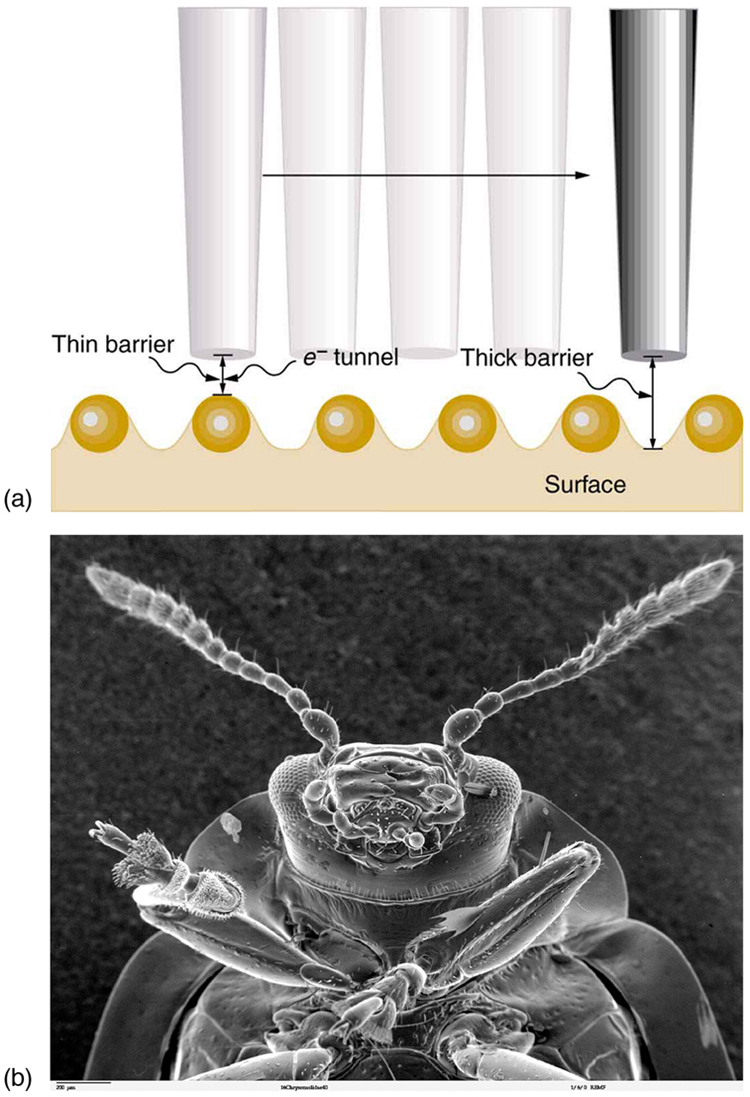PhET Explorations: Quantum Tunneling and Wave Packets

Watch quantum “particles” tunnel through barriers. Explore the properties of the wave functions that describe these particles. Click to open media in new browser.

### Section Summary

• Tunneling is a quantum mechanical process of potential energy barrier penetration. The concept was first applied to explaindecay, but tunneling is found to occur in other quantum mechanical systems.

### Conceptual Questions

A physics student caught breaking conservation laws is imprisoned. She leans against the cell wall hoping to tunnel out quantum mechanically. Explain why her chances are negligible. (This is so in any classical situation.)

When a nucleusdecays, does theparticle move continuously from inside the nucleus to outside? That is, does it travel each point along an imaginary line from inside to out? Explain.

### Problems-Exercises

Derive an approximate relationship between the energy ofdecay and half-life using the following data. It may be useful to graph the log of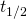against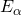to find some straight-line relationship.

Energy and Half-Life forDecay
Nuclide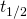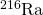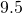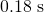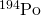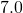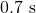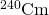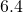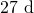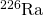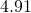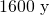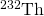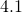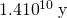Integrated Concepts

A 2.00-T magnetic field is applied perpendicular to the path of charged particles in a bubble chamber. What is the radius of curvature of the path of a 10 MeV proton in this field? Neglect any slowing along its path.

22.8 cm

(a) Write the decay equation for thedecay of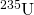. (b) What energy is released in this decay? The mass of the daughter nuclide is 231.036298 u. (c) Assuming the residual nucleus is formed in its ground state, how much energy goes to theparticle?

(a)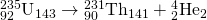(b) 4.679 MeV

(c) 4.599 MeV

Unreasonable Results

The relatively scarce naturally occurring calcium isotope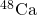has a half-life of about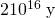. (a) A small sample of this isotope is labeled as having an activity of 1.0 Ci. What is the mass of thein the sample? (b) What is unreasonable about this result? (c) What assumption is responsible?

Unreasonable Results

A physicist scatters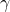rays from a substance and sees evidence of a nucleus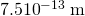in radius. (a) Find the atomic mass of such a nucleus. (b) What is unreasonable about this result? (c) What is unreasonable about the assumption?

a)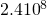u

(b) The greatest known atomic masses are about 260. This result found in (a) is extremely large.

(c) The assumed radius is much too large to be reasonable.

Unreasonable Results

A frazzled theoretical physicist reckons that all conservation laws are obeyed in the decay of a proton into a neutron, positron, and neutrino (as in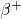decay of a nucleus) and sends a paper to a journal to announce the reaction as a possible end of the universe due to the spontaneous decay of protons. (a) What energy is released in this decay? (b) What is unreasonable about this result? (c) What assumption is responsible?

(a)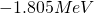(b) Negative energy implies energy input is necessary and the reaction cannot be spontaneous.

(c) Although all conversation laws are obeyed, energy must be supplied, so the assumption of spontaneous decay is incorrect.

Consider the decay of radioactive substances in the Earth’s interior. The energy emitted is converted to thermal energy that reaches the earth’s surface and is radiated away into cold dark space. Construct a problem in which you estimate the activity in a cubic meter of earth rock? And then calculate the power generated. Calculate how much power must cross each square meter of the Earth’s surface if the power is dissipated at the same rate as it is generated. Among the things to consider are the activity per cubic meter, the energy per decay, and the size of the Earth.

### Glossary

barrier penetration
quantum mechanical effect whereby a particle has a nonzero probability to cross through a potential energy barrier despite not having sufficient energy to pass over the barrier; also called quantum mechanical tunneling
quantum mechanical tunneling
quantum mechanical effect whereby a particle has a nonzero probability to cross through a potential energy barrier despite not having sufficient energy to pass over the barrier; also called barrier penetration
tunneling
a quantum mechanical process of potential energy barrier penetration